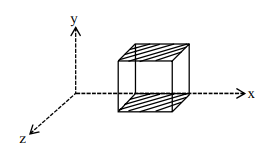# A cube is placed inside an electric field,`
Question:

A cube is placed inside an electric field, $\overrightarrow{\mathrm{E}}=150 \mathrm{y}^{2} \hat{\mathrm{j}}$. The side of the cube is $0.5 \mathrm{~m}$ and is placed in the field as shown in the given figure. The charge inside the cube is:1. $3.8 \times 10^{-11} \mathrm{C}$

2. $8.3 \times 10^{-11} \mathrm{C}$

3. $3.8 \times 10^{-12} \mathrm{C}$

4. $8.3 \times 10^{-12} \mathrm{C}$

Correct Option: , 2

Solution:

As electric field is in y-direction so electric flux is

only due to top and bottom surface

Bottom surface $y=0$

$\Rightarrow \mathrm{E}=0 \Rightarrow \phi=0$

Now flux $\phi=\mathrm{EA}=\frac{150}{4}(.5)^{2}=\frac{150}{16}$

By Gauss's law $\phi=\frac{Q_{\text {in }}}{\epsilon_{0}}$

$\frac{150}{16}=\frac{\mathrm{Q}_{\text {in }}}{\epsilon_{0}}$

$\mathrm{Q}_{\mathrm{in}}=\frac{150}{16} \times 8.85 \times 10^{-12}=8.3 \times 10^{-11} \mathrm{C}$

Option (2)